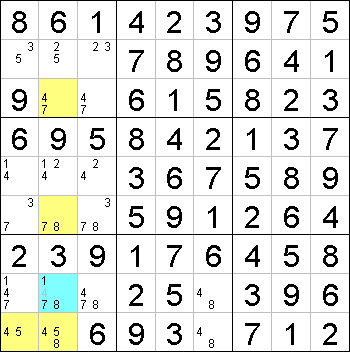Mirrored from Sudopedia, the Free Sudoku Reference Guide

# Subset Counting

Subset Counting is a highly advanced solving technique that requires a lot of searching, counting, testing and calculating. Thus, it is not a technique suitable for your everyday Sudoku, but one that should be reserved for the extremely difficult puzzles.

Subset Counting can replicate XY-Wing, XYZ-Wing, WXYZ-Wing, Aligned Pair Exclusion, Aligned Triple Exclusion and ALS-XZ moves, but there may be broader applications which have not been investigated yet.

The theorem behind Subset Counting is sometimes called Extended Subset Principle. Hence, the terms "Subset Counting" and "Extended Subset Principle" may be used interchangeably.

## How it works

Select a group of cells which you would like to investigate. The best choice - for now - is a group of cells which forms an Almost Locked Set. These offer the best chance to produce results.

For each digit, count the number of ways it can be placed in the selected cells. There is no need to look at interaction between digits. Just test each digit on its own. Now calculate the sum of these counts. When it is less than the number of selected cells, you've done something wrong, because that cannot happen in a valid puzzle. When it is equal to the number of selected cells, you've overlooked something. There is only one way to complete these cells.

Continue with this technique when the total count is 1 or 2 more than the number of selected cells, and there is 1 digit that has a count greater than this surplus.

Now check the grid to see if there is a candidate that can reduce the total count below the number of selected cells. Focus your attention on peers of the cells for which removing the candidates for the same digit will reduce the total count below the set size. You can eliminate each candidate that would cause this to happen.

## ExampleThis example illustrates how Subset Counting works. It works on any selected set of cells so long you can make eliminations. We start off by selecting the 4 cells marked yellow. For now, let us ignore how these cells were selected. Note that the selected cells have the digits 4, 5, 7 and 8 as their candidates. We now determine the maximum number of times each of these digits can be placed in the selected cells: The digit 4 can be placed in a maximum of 2 cells, in r3c2 and r9c1. The digit 5 can be placed in a maximum of 1 cell, in r9c1 or r9c2. The digit 7 can be placed in a maximum of 1 cell, in r3c2 or r6c2. The digit 8 can be placed in a maximum of 1 cell, in r6c2 or r9c2. Summing up the maximum number of placements, we obtain 2 + 1 + 1 + 1 = 5. Now suppose the blue cell contains the digit 4. This eliminates the digit 4 from r3c2, r9c1 and r9c2, so the maximum number of cells the digit 4 can be placed drops to 0, i.e. a decrease of 2. Thus the the sum is changed to 5 - 2 = 3. This means that you can only place a maximum of 3 digits into these 4 cells, which is impossible. This contradiction means that the blue cell cannot contain the digit 4, so we can eliminate it. On the other hand, we cannot eliminate the digit 4 from r5c2 yet, because the decrease of sum is only 1, which equates to the number of selected cells and does not cause a contradiction. Actually, the selected cells form a WXYZ-Wing. The WXYZ-Wing gives the same elimination.

## Remarks

The above example illustrates both the power and difficulty in applying the Subset Counting technique. The difficulty lies in how to select cells for the counting. However, the power of this technique makes it quite possible to devise other easier to use techniques, with the correctness of these easier techniques "proven immediately from the Extended Subset Principle".